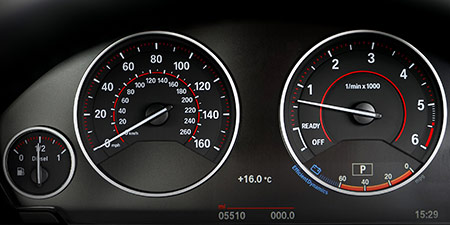# Speed of sound to Miles per second (mi/s)

Units of speed ﹣ Converter Speed of sound to Miles per second

Here you can convert the Speed unit Speed of sound into the unit Miles per second and vice versa you can convert Miles per second into Speed of sound. By clicking the "Swap units" icon, you will always obtain the desired conversion in the calculation result. With the following calculator you can also calculate any other Speed unit.

## Info about "Speed of sound"

Definition: If an object travels at the speed of sound in air at 20 degrees Celsius for one second, it travels a distance of 343 meters per second. Actually, it is equivalent to 1.234,8 km/h.

The speed of sound is often illustrated by an example of a thunderstorm: if lightning strikes within 3 seconds of the thunder sound, then the thunderstorm is about 1 kilometer away. Another example, in a 100-meter sprint, the starting shot is fired directly next to the starting blocks. Imagine, if the start shot was fired at the finish line, it would take about 0.3 seconds of time to hear the shot in the start blocks.

## Info about "Miles per second"

The length unit "mile" is commonly used in the Anglo-American countries, where speeds are represented in miles (instead of kilometers) per time unit and it should not be confused sea mile.

The units are defined as follows: An object moving at 1 "mph" per hour will cover a distance of one mile. Therefore, 1 mile is equivalent to 1.609344 kilometers.

## Formula for the conversion of Speed of sound to Miles per second (mi/s) and vice versa

The calculation from Speed of sound to Miles per second shall be made using the following conversion formula:

Conversion formula Speed of sound to Miles per second

Determine the number of Miles per second from Speed of sound

Speed of sound × 0.21313031893741

## Formula for the conversion of Miles per second (mi/s) to Speed of sound

The calculation from Miles per second to Speed of sound shall be made using the following conversion formula:

Conversion formula Miles per second to Speed of sound

Determine the number of Speed of sound from Miles per second

Miles per second × 4.6919650145773

## Overview table : How many Speed of sound are in a Miles per second ?

Speed of sound ⇒ Miles per second mi/s
0.01   are  0.00213 mi/s
0.02   are  0.00426 mi/s
0.03   are  0.00639 mi/s
0.04   are  0.00852 mi/s
0.05   are  0.01065 mi/s
0.06   are  0.01278 mi/s
0.07   are  0.01491 mi/s
0.08   are  0.01705 mi/s
0.09   are  0.01918 mi/s
0.10   are  0.02131 mi/s
0.20   are  0.04262 mi/s
0.30   are  0.06393 mi/s
0.40   are  0.08525 mi/s
0.50   are  0.10656 mi/s
0.60   are  0.12787 mi/s
0.70   are  0.14919 mi/s
0.80   are  0.17050 mi/s
0.90   are  0.19181 mi/s
1   corresponds to  0.21313 mi/s
2   are  0.42626 mi/s
3   are  0.63939 mi/s
4   are  0.85252 mi/s
5   are  1.06565 mi/s
6   are  1.27878 mi/s
7   are  1.49191 mi/s
8   are  1.70504 mi/s
9   are  1.91817 mi/s
10   are  2.13130 mi/s
20   are  4.26260 mi/s
30   are  6.39391 mi/s
40   are  8.52521 mi/s
50   are  10.65651 mi/s
60   are  12.78781 mi/s
70   are  14.91912 mi/s
80   are  17.05042 mi/s
90   are  19.18172 mi/s
100   are  21.31303 mi/s
200   are  42.62606 mi/s
300   are  63.93909 mi/s
400   are  85.25212 mi/s
500   are  106.56515 mi/s
600   are  127.87819 mi/s
700   are  149.19122 mi/s
800   are  170.50425 mi/s
900   are  191.81728 mi/s
1 000   are  213.13031 mi/s

## Overview table : How many Miles per second are in a Speed of sound ?

Miles per second mi/s ⇒ Speed of sound
0.01 mi/s  are  0.04692
0.02 mi/s  are  0.09383
0.03 mi/s  are  0.14075
0.04 mi/s  are  0.18767
0.05 mi/s  are  0.23459
0.06 mi/s  are  0.28151
0.07 mi/s  are  0.32843
0.08 mi/s  are  0.37535
0.09 mi/s  are  0.42227
0.10 mi/s  are  0.46919
0.20 mi/s  are  0.93839
0.30 mi/s  are  1.40759
0.40 mi/s  are  1.87678
0.50 mi/s  are  2.34598
0.60 mi/s  are  2.81517
0.70 mi/s  are  3.28437
0.80 mi/s  are  3.75357
0.90 mi/s  are  4.22276
1 mi/s  corresponds to  4.69196
2 mi/s  are  9.38393
3 mi/s  are  14.07589
4 mi/s  are  18.76786
5 mi/s  are  23.45982
6 mi/s  are  28.15179
7 mi/s  are  32.84375
8 mi/s  are  37.53572
9 mi/s  are  42.22768
10 mi/s  are  46.91965
20 mi/s  are  93.83930
30 mi/s  are  140.75895
40 mi/s  are  187.67860
50 mi/s  are  234.59825
60 mi/s  are  281.51790
70 mi/s  are  328.43755
80 mi/s  are  375.35720
90 mi/s  are  422.27685
100 mi/s  are  469.19650
200 mi/s  are  938.39300
300 mi/s  are  1 407.58950
400 mi/s  are  1 876.78600
500 mi/s  are  2 345.98250
600 mi/s  are  2 815.17900
700 mi/s  are  3 284.37551
800 mi/s  are  3 753.57201
900 mi/s  are  4 222.76851
1 000 mi/s  are  4 691.96501

## Source information

As source for the information in the 'Units of speed' category, we have used in particular:

## Last update on February 19, 2023

The pages of the 'Units of speed' category were last editorially reviewed by Stefan Banse on February 19, 2023. They all correspond to the current status.

### Previous changes on October 9, 2021

• November 9, 2020: Publication of the Speed converter
• Editorial revision of all texts in this category